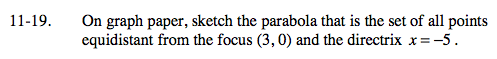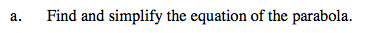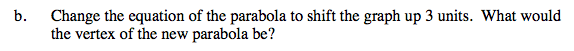### Home > A2C > Chapter 11 > Lesson 11.1.2 > Problem11-19

11-19.Find the expressions for the distance from the directrix to the point (x, y) and the distance from the focus to the same point, then set both of them equal to each other.

$\sqrt{(x - 3)^2 + y^2} = x + 5$

Now simplify.Subtract 3 from y before squaring to shift the graph up 3 units.